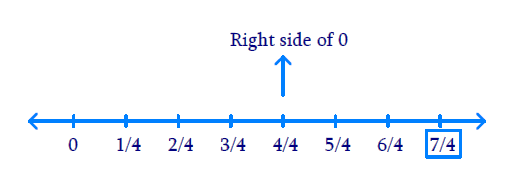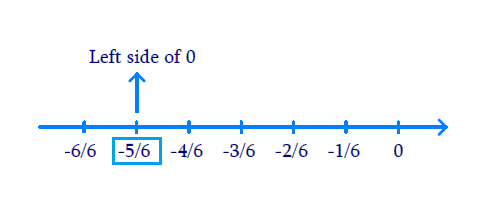# Ex.1.2 Q1 Rational Numbers Solution - NCERT Maths Class 8

Go back to  'Ex.1.2'

## Question

Represent these numbers on the number line.

(i) \begin{align}\;\frac{7}{4}\end{align}

(ii) \begin{align}\;\frac{{ - 5}}{6}\end{align}

Video Solution
Rational Numbers
Ex 1.2 | Question 1

## Text Solution

Reasoning:

The positive numbers are on the right of $$0$$ and negative numbers are represented on the left of $$0$$ in the number line. The denominator of the rational number indicates the number of equal parts into which the first unit has to be divided whereas the numerator indicates as to how many of these parts are to be taken into consideration.

Steps:

\begin{align}\frac{7}{4}\end{align} The first unit has to be divided by $$4$$ parts. We make $$7$$ markings of distance

\begin{align}\frac{1}{4}\end{align} Each on the right of $$0$$ and starting from $$0.$$ The seventh marking represents \begin{align}\frac{7}{4}\end{align}(i) \begin{align}\frac{{ - 5}}{6}\end{align} The first unit has to be divided into $$5$$ parts. We make $$5$$ markings of distance \begin{align}\frac{1}{6}\end{align} each on the left of $$0$$ and starting from $$0.$$

The fifth marking represents \begin{align}\frac{5}{6}\end{align} left side of $$0.$$Learn from the best math teachers and top your exams

• Live one on one classroom and doubt clearing
• Practice worksheets in and after class for conceptual clarity
• Personalized curriculum to keep up with school